# Area of a Circle

Go back to  'Circles'

 1 What is a Circle? 2 What is Area of Circle 3 Area of Circle Example & Formulas 4 Derivation of Area of a Circle 5 Surface Area of Circle Formula 6 Important Notes on Area of Circle 7 Real-World Examples 8 Solved Examples 9 Thinking out of the Box! 10 Practice Questions 11 Challenging Questions on Area of Circle 12 Maths Olympiad Sample Papers 13 Frequently Asked Questions (FAQs) 14 FREE Worksheets on Area of Circle

We at Cuemath believe that Math is a life skill. Our Math Experts focus on the “Why” behind the “What.” Students can explore from a huge range of interactive worksheets, visuals, simulations, practice tests, and more to understand a concept in depth.

Book a FREE trial class today! and experience Cuemath's LIVE Online Class with your child.

## What is a Circle?

A Circle is a round closed figure where all its boundary points are equidistant from a fixed point, the centre.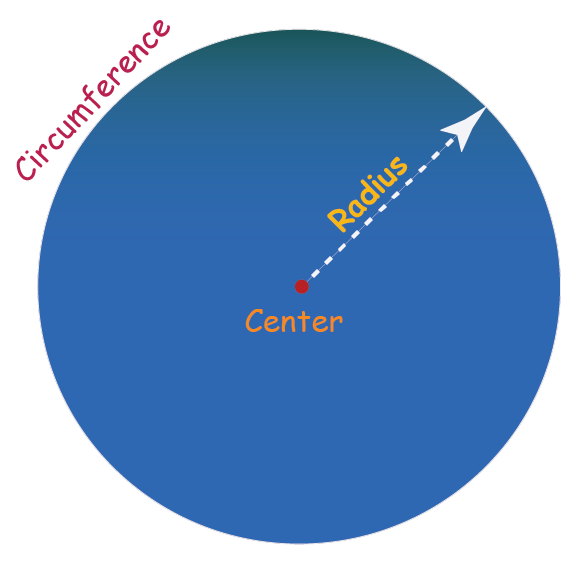The distance from the centre to a point on the boundary is called the radius of a circle. It is represented by the letter "$$r$$"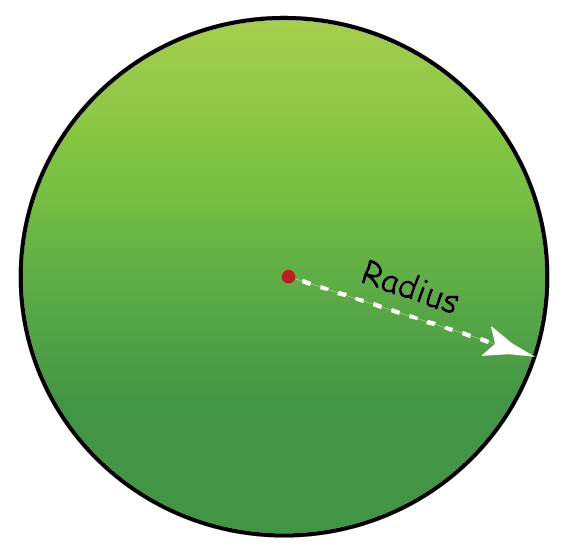### Diameter

A straight line which passes through the centre and its endpoints lie on the circle is called the diameter of a circle. It is represented by the letter "$$d$$"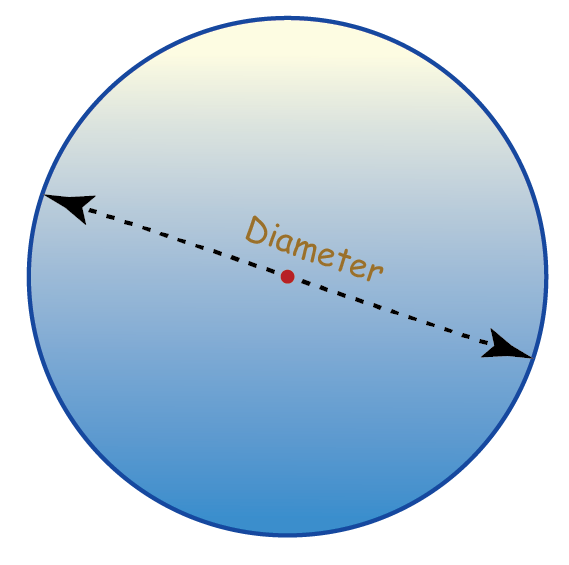The diameter of a circle is twice its radius.

 $$\text{Diameter } \left(d\right)$$ $$= 2 \times \text{ Radius} \left(r\right)$$

### Circumference

The total length of the boundary is called the circumference of a circle.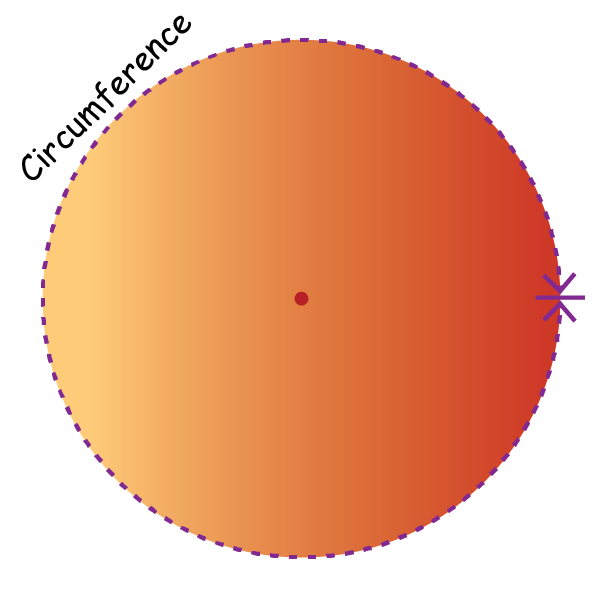$$\text{Circumference of a Circle}$$ $$= 2 \pi r$$

where $$r$$ is the radius of the circle and $$\text{Pi} - \pi$$  is the mathematical constant whose value is approximated to $$3.14$$  or $$\frac{22}{7}$$

## What is Area of Circle

The area of a circle is the amount of space enclosed within the boundary of a circle.

In the below figures, the colored region within the boundary of the circle is the area occupied by the circle.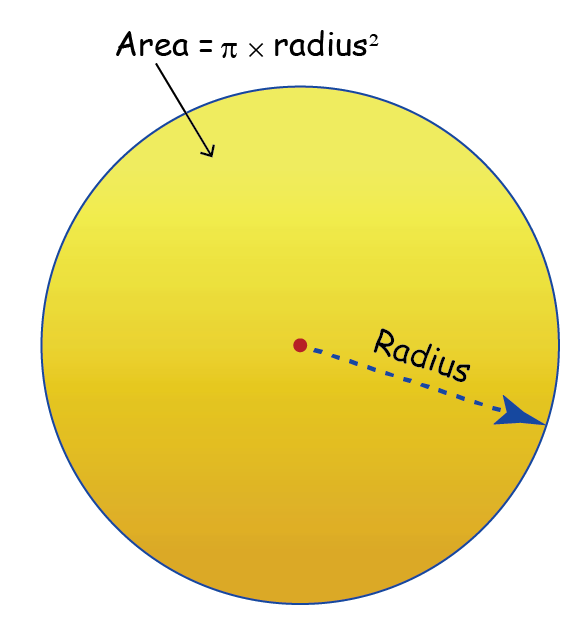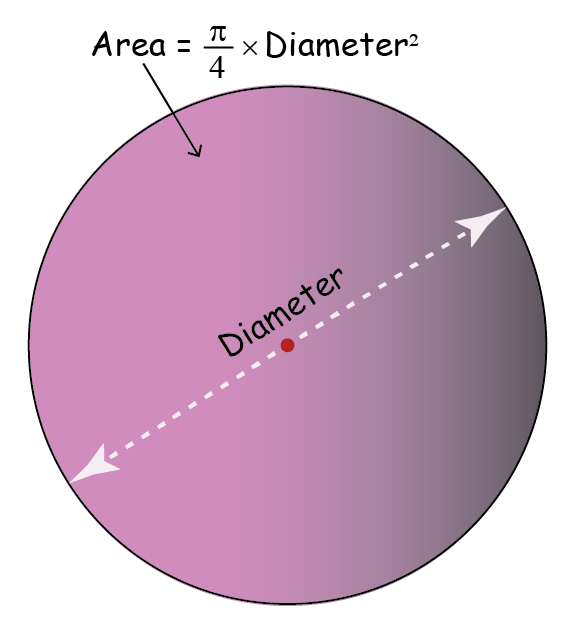## Area of Circle Example & Formulas

• Area of circle formula in terms of the radius is:
 $$\text{Area of a Circle} = \pi \text{r}^2$$
•  Area of the circle formula in terms of the diameter is:
 \begin{align} \text{Area of a Circle} = \!\frac{\pi}{4} \!\times\! \text{d}^2\end{align}

$$\text{r}$$ is the radius of the circle and $$\text{d}$$ is the diameter of the circle

The value of $$\pi \approx \frac{22}{7}$$

Improve your knowledge on Area of a Circle with examples from our experts in the Solved Examples section which has few sums based on this formula.

## Derivation of Area of a Circle

Before we give the formula, let's first understand how the formula for area of a circle is derived.

Here's a simple simulation for you to interact with along with explanations.

• As we can see in the simulation above, the circle can be cut into a triangle with the radius being the height of the triangle and the perimeter as its base which is
$2 \pi r$
• We know that the area of the triangle is found by multiplying its base by the height, and then dividing by 2, which is
\begin{align}\left(\frac{1}{2}\right) \times {2} \pi r \times r\end{align}
• Therefore, the area of the circle is $$\pi {r^2}$$
 $$\text{Area of a Circle}$$ $$= \pi r^2$$

where $$r$$ is the radius of the circle and the value of $$\pi \approx \frac{22}{7}$$

## Surface Area of Circle Formula

Surface area is the area occupied by the surface of a 3-D shape. The surface of a sphere will be circular in shape.

A circle is a simple plane 2-Dimensional shape. The total area occupied by the circle is the surface area of the circle

Surface Area Formula of a circle is:

 $$\text{Surface Area of a Circle}$$ $$= \pi r^2$$

where $$r$$ is the radius of the circle and the value of $$\pi \approx \frac{22}{7}$$

Circles
grade 9 | Questions Set 2
Circles
grade 9 | Questions Set 1
Circles